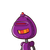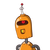# 16] There are 25 boys and 15 girls in a class. Calculate the ratio of: Thenumber of girls to the number of boys.1. 7:5

16] There are 25 boys and 15 girls in a class. Calculate the ratio of: The
number of girls to the number of boys.
1. 7:5
2. 3:5
3. 5:3
4. 1:5​

### 2 thoughts on “16] There are 25 boys and 15 girls in a class. Calculate the ratio of: The<br />number of girls to the number of boys.<br />1. 7:5”

1.Step-by-step explanation: 15 / 25 = 3/5

3: 5 is the answer for above question

2.